# NCERT Solutions for Chapter 7 Can You See The Pattern? Class 5 Maths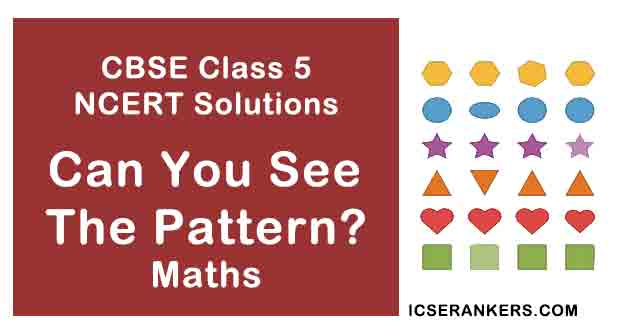Can You See The Pattern? Questions and Answers

 Chapter Name Can You See The Pattern? NCERT Solutions Class CBSE Class 5 Textbook Name Maths Related Readings NCERT Solutions for Class 5NCERT Solutions for Class 5 Maths Summary of Can You See The Pattern?

Page No 99:

Question 1: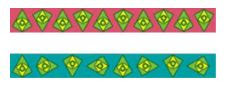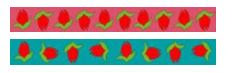Page No 100:

Question 1: What should come next?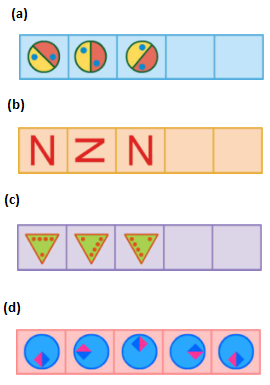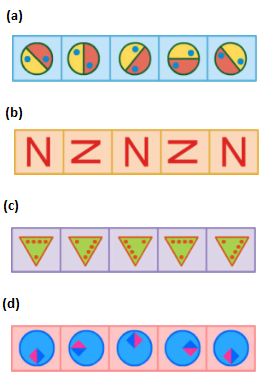Page No 101:

Question 1: See this pattern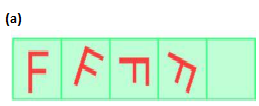The rule of the pattern is â€ turning by 45° each time. Which will be the next? Tick () the right one.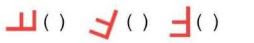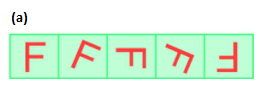Following the pattern, the next figure will be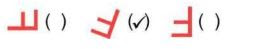Question 2: Using the same rule take it forward till you get back to what you started with.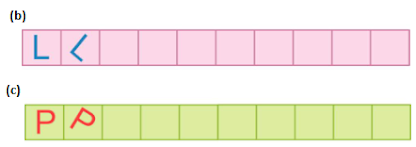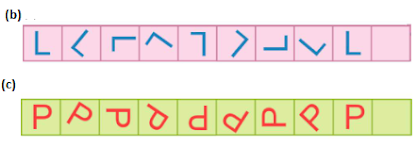Page No 102:

Question 1: Some patterns are given below on the left side of the red line. For each patter, write the rule. Then choose what comes next from the right side of the line and tick () it.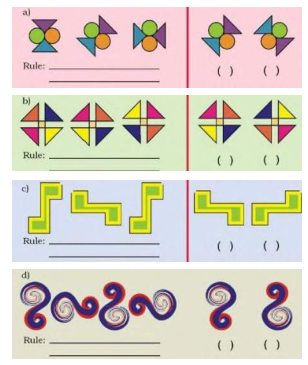(a) Rule: Turn by 45 °each time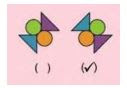(b) Rule: Turn by 90° each time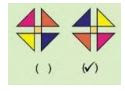(c) Rule: Turn by 90° each time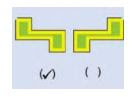(d) Rule: Turn by 90° each timePage No 103:

Question 1: Look for a Pattern Mark that picture which is breaking the rule. Also correct it.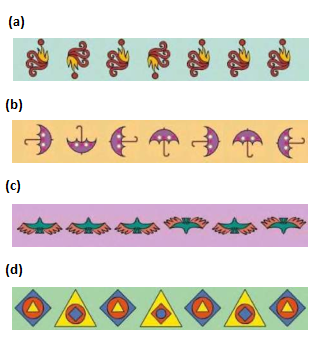(a)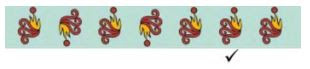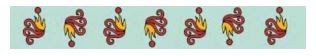(b)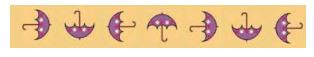(c)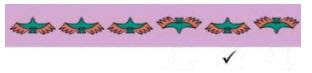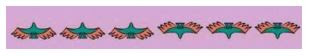(d)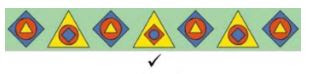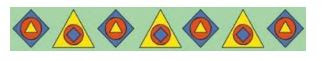Question 2:

• Fill this square using all the numbers from 46 to 54. Rule: The total of each line is 150.

 49 46 52 47

• Fill this square using all the numbers from 21 to 29. Rule: The total of each side is 75.

 25

•

 53 48 49 46 50 54 51 52 47

 24 23 28 29 25 21 22 27 26

Page No 104:

Question 1: Look at the patterns of number in hexagons. Each side has 2 circle and 1 box.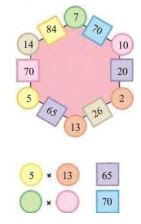Look at the number 65 in the box. Which are the circles next to it? Can you see how the rule works?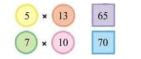Circles having numbers 5 and 13 are next to the number 65. In this rule, both the numbers in the circles are multiplied to get the number in the box.

Question 2: Use the same rule to fill the hexagons below.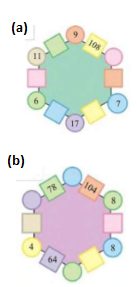Now you also make your own magic hexagons.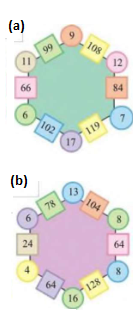Disclaimer: The answer may vary from student to student, based on his/her observation. It is highly recommended that the students prepare the answer on their own.

Page No 105:

Question 1: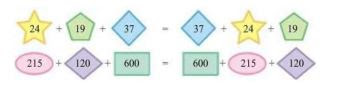Are they equal?

Yes, both of them are equal because we are adding same numbers on both the sides, only their order will change.

24+19+37=80, 37 +24+ 19 = 80

215 +120+600=935, 600 +215+ 120=935

Order does not matter in addition.

Question 2: Fill in the blank spaces in the same way.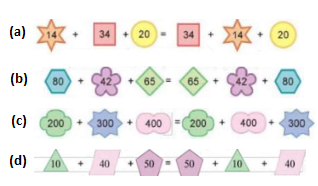Disclaimer: The answer in part (d) of the question varies from student to student. The answer provided here is only for reference.

Question 3: Now, look at this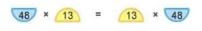Check if it is true or not.

48 × 13 = 624

13 × 48 = 624 Their multiplication is equal. So, it is true.

Page No 106:

Question 1: Try and change these numbers into special numbers — (a) 28 (b) 132 (c) 273

(a) Take number 28, turn it in the reverse order to get 82. Add them together: 28 +82 = 110 But this is not a special number because when we reverse its order, we get 011. Now, we add: 110 + 011 = 121 When we reverse its order, we get 121. Thus, it is a special number.

(b) Take the number 132, turn it in the reverse order to get 231. Add them together: 132 +231 = 363 When we reverse its order, we get 363. Thus, we get the special number as required.

(c) Take the number 273, turn it in the reverse order to get 372 Add them together: 273 +372 = 645 But this is not a special number because when we reverse its order, we get 546. Now, if we subtract them, we get 645 546=99 When we reverse its order, we get 99, which is a special number.

Question 2: NO LEMONS NO MELON STEP NOT ON PETS

Did you notice that it reads the same from both sides - right to left and left to right? Now try and use words in a special way.

MADAM, EYE, RACE CAR, A SANTA AT NASA

Disclaimer: The answer may vary from student to student. It is highly recommended that the students prepare the answer on their own. The answer provided here is only for reference.

Page No 107:

Question 1: Look at the calendar below. Let us mark a 3 x 3 box (9 dates) on the calendar and see some magic.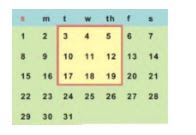Take the smallest number 3

= 11

Multiply it by 9 x 9

Total 99

Now you choose any 3 × 3 box from a calendar and find the total in the same way.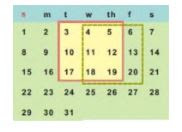Take the smallest number = 4

Adding 8 to it, we get 12.

Multiplying it by 9, we get 12 x 9= 108

So, the sum of all the numbers in the selected box is 108.

Page No 108:

Question 1: Take any number. Now multiply it by 2, 3, 4……… at every step. also add 3 to it at each step. Look at the difference in the answer. Is it the same at every step?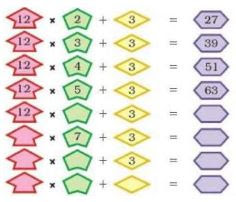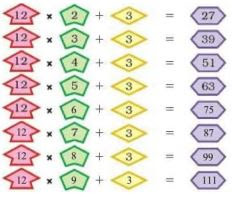Difference between 39 27 = 12 Difference between 51 39 = 12

Difference between 63 51 = 12 Difference between 75 63 = 12

Difference between 87 75= 12 Difference between 99 87 = 12 Thus, the difference is same at all the steps.

Question 2: Look at the numbers below. Look for the pattern. Can you take it forward?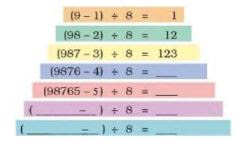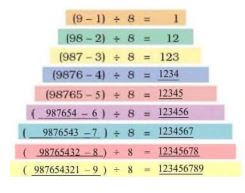Page No 109:

Question 1: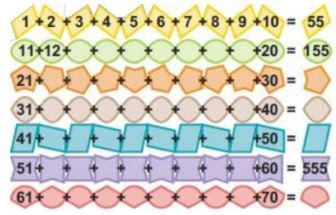Did you notice some pattern in the answers?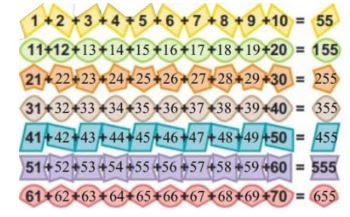In each succeeding pattern, the final sum is increased by 100.

Question 2: Take the first two odd numbers. Now add them, see what you get. Now, at every step, add the next odd number.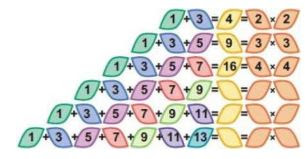How far can you go on?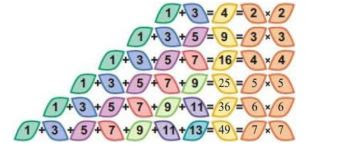As there are uncountable or infinite numbers, this pattern will never come to an end.

Page No 110:

Question 1: Ask your friend - Write down your age. Add 5 to it. Multiply the sum by 2. Subtract 10 from it. Next divide it by 2. What do you get? Is your friend surprised?

Let the age of my friend be 9 years.

Adding 5 to it, we get 9 + 5 = 14

Multiplying this sum by 2, we get 14 x 2 = 28

Subtracting 10, we get 28 -10 = 18

Dividing it by 2, we get 18/2 = 9

After doing all the operations, we again get the age of our friend's age as the final answer.

Disclaimer: The answer may vary from student to student based on his/her observation. The answer provided here is only for reference.

Page No 111:

Question 1: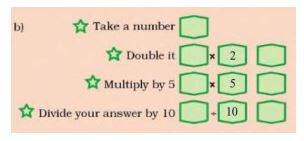Disclaimer: The answer may vary from student to student. The answers provided here are only for reference.

Question 2: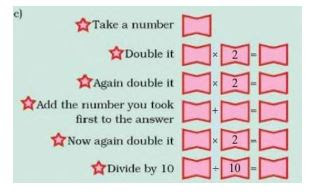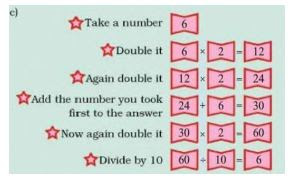Disclaimer: The answer may vary from student to student. The answers provided here are only for reference.

Question 3: Look at this pattern of numbers and take it forward.

1              = 1 x 1

121         = 11 × 11

12321     = 111 × 111

1234321 = ?

1                         = 1 × 1

121                     = 11 x 11

12321                 = 111 x 111

1234321             = 1111 × 1111

123454321         = 11111 × 11111

12345654321     = 111111 x 111111